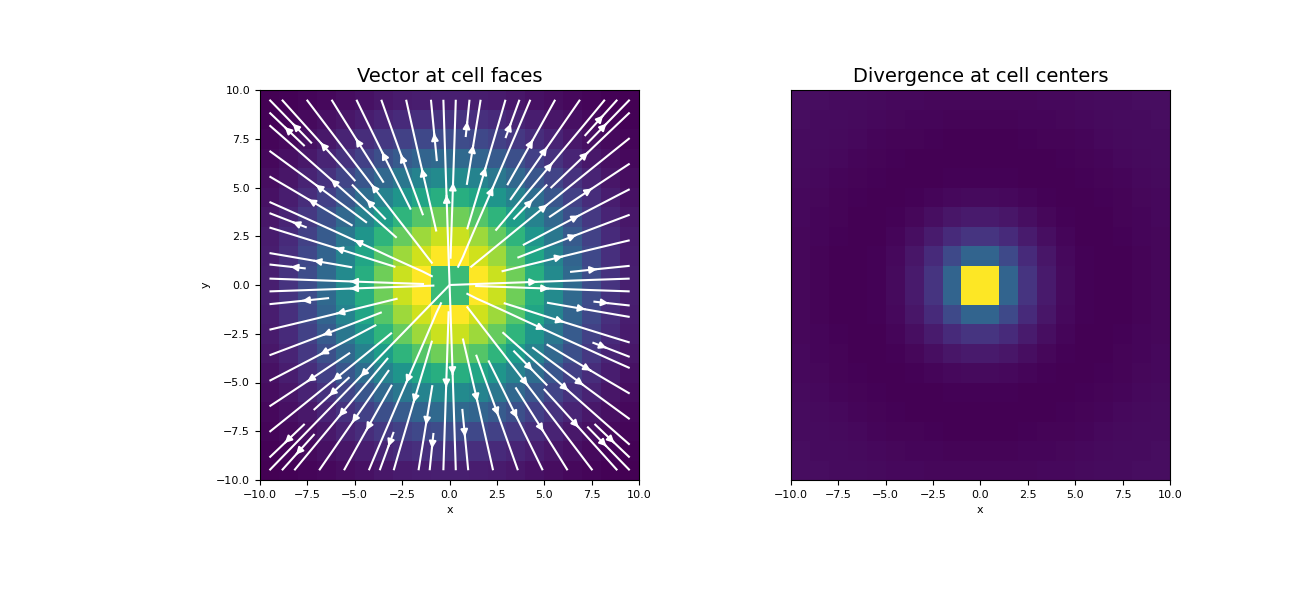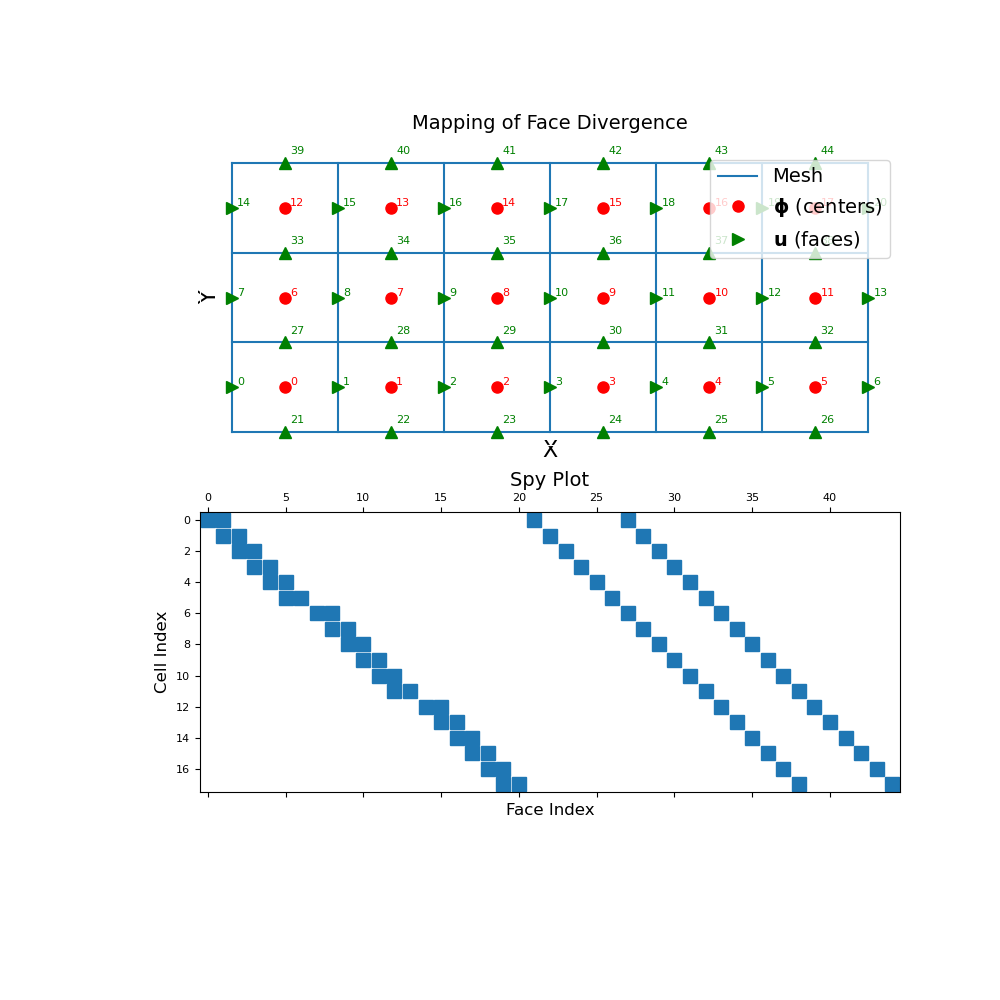# discretize.operators.DiffOperators.face_divergence¶

property DiffOperators.face_divergence

Face divergence operator (faces to cell-centres)

This property constructs the 2nd order numerical divergence operator that maps from faces to cell centers. The operator is a sparse matrix $$\mathbf{D_f}$$ that can be applied as a matrix-vector product to a discrete vector $$\mathbf{u}$$ that lives on mesh faces; i.e.:

div_u = Df @ u


Once constructed, the operator is stored permanently as a property of the mesh. See notes for additional details.

Returns
(n_cells, n_faces) scipy.sparse.csr_matrix

The numerical divergence operator from faces to cell centers

Notes

In continuous space, the divergence operator is defined as:

$\phi = \nabla \cdot \vec{u} = \frac{\partial u_x}{\partial x} + \frac{\partial u_y}{\partial y} + \frac{\partial u_z}{\partial z}$

Where $$\mathbf{u}$$ is the discrete representation of the continuous variable $$\vec{u}$$ on cell faces and $$\boldsymbol{\phi}$$ is the discrete representation of $$\phi$$ at cell centers, face_divergence constructs a discrete linear operator $$\mathbf{D_f}$$ such that:

$\boldsymbol{\phi} = \mathbf{D_f \, u}$

For each cell, the computation of the face divergence can be expressed according to the integral form below. For cell $$i$$ whose corresponding faces are indexed as a subset $$K$$ from the set of all mesh faces:

$\phi_i = \frac{1}{V_i} \sum_{k \in K} A_k \, \vec{u}_k \cdot \hat{n}_k$

where $$V_i$$ is the volume of cell $$i$$, $$A_k$$ is the surface area of face k, $$\vec{u}_k$$ is the value of $$\vec{u}$$ on face k, and $$\hat{n}_k$$ represents the outward normal vector of face k for cell i.

Examples

Below, we demonstrate 1) how to apply the face divergence operator to a discrete vector and 2) the mapping of the face divergence operator and its sparsity. Our example is carried out on a 2D mesh but it can be done equivalently for a 3D mesh.

We start by importing the necessary packages and modules.

>>> from discretize import TensorMesh
>>> import numpy as np
>>> import matplotlib.pyplot as plt
>>> import matplotlib as mpl


Define a 2D mesh

>>> h = np.ones(20)
>>> mesh = TensorMesh([h, h], "CC")


Create a discrete vector on mesh faces

>>> faces_x = mesh.faces_x
>>> faces_y = mesh.faces_y
>>> ux = (faces_x[:, 0] / np.sqrt(np.sum(faces_x ** 2, axis=1))) * np.exp(
...     -(faces_x[:, 0] ** 2 + faces_x[:, 1] ** 2) / 6 ** 2
... )
>>> uy = (faces_y[:, 1] / np.sqrt(np.sum(faces_y ** 2, axis=1))) * np.exp(
...     -(faces_y[:, 0] ** 2 + faces_y[:, 1] ** 2) / 6 ** 2
... )
>>> u = np.r_[ux, uy]


Construct the divergence operator and apply to face-vector

>>> Df = mesh.face_divergence
>>> div_u = Df @ u


Plot the original face-vector and its divergence

Expand to show scripting for plot
>>> fig = plt.figure(figsize=(13, 6))
>>> mesh.plot_image(
...     u, ax=ax1, v_type="F", view="vec", stream_opts={"color": "w", "density": 1.0}
... )
>>> ax1.set_title("Vector at cell faces", fontsize=14)
>>> mesh.plot_image(div_u, ax=ax2)
>>> ax2.set_yticks([])
>>> ax2.set_ylabel("")
>>> ax2.set_title("Divergence at cell centers", fontsize=14)
>>> plt.show()The discrete divergence operator is a sparse matrix that maps from faces to cell centers. To demonstrate this, we construct a small 2D mesh. We then show the ordering of the elements in the original discrete quantity $$\mathbf{u}$$ and its discrete divergence $$\boldsymbol{\phi}$$ as well as a spy plot.

Expand to show scripting for plot
>>> mesh = TensorMesh([[(1, 6)], [(1, 3)]])
>>> fig = plt.figure(figsize=(10, 10))
>>> mesh.plot_grid(ax=ax1)
>>> ax1.plot(
...     mesh.cell_centers[:, 0], mesh.cell_centers[:, 1], "ro", markersize=8
... )
>>> for ii, loc in zip(range(mesh.nC), mesh.cell_centers):
...     ax1.text(loc+0.05, loc+0.02, "{0:d}".format(ii), color="r")
>>> ax1.plot(
...     mesh.faces_x[:, 0], mesh.faces_x[:, 1], "g>", markersize=8
... )
>>> for ii, loc in zip(range(mesh.nFx), mesh.faces_x):
...     ax1.text(loc+0.05, loc+0.02, "{0:d}".format(ii), color="g")
>>> ax1.plot(
...     mesh.faces_y[:, 0], mesh.faces_y[:, 1], "g^", markersize=8
... )
>>> for ii, loc in zip(range(mesh.nFy), mesh.faces_y):
...     ax1.text(loc + 0.05, loc + 0.1, "{0:d}".format((ii + mesh.nFx)), color="g")

>>> ax1.set_xticks([])
>>> ax1.set_yticks([])
>>> ax1.spines['bottom'].set_color('white')
>>> ax1.spines['top'].set_color('white')
>>> ax1.spines['left'].set_color('white')
>>> ax1.spines['right'].set_color('white')
>>> ax1.set_title("Mapping of Face Divergence", fontsize=14, pad=15)
>>> ax1.legend(
...     ['Mesh', '$\mathbf{\phi}$ (centers)', '$\mathbf{u}$ (faces)'],
...     loc='upper right', fontsize=14
... )